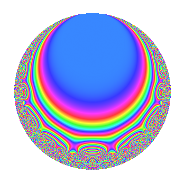# Properties

 Label 18.5.dLevel 18 Weight 5 Character orbit d Rep. character $$\chi_{18}(5,\cdot)$$ Character field $$\Q(\zeta_{6})$$ Dimension 8 Newforms 1 Sturm bound 15 Trace bound 0

# Related objects

## Defining parameters

 Level: $$N$$ = $$18 = 2 \cdot 3^{2}$$ Weight: $$k$$ = $$5$$ Character orbit: $$[\chi]$$ = 18.d (of order $$6$$ and degree $$2$$) Character conductor: $$\operatorname{cond}(\chi)$$ = $$9$$ Character field: $$\Q(\zeta_{6})$$ Newforms: $$1$$ Sturm bound: $$15$$ Trace bound: $$0$$

## Dimensions

The following table gives the dimensions of various subspaces of $$M_{5}(18, [\chi])$$.

Total New Old
Modular forms 28 8 20
Cusp forms 20 8 12
Eisenstein series 8 0 8

## Trace form

 $$8q$$ $$\mathstrut +\mathstrut 6q^{3}$$ $$\mathstrut +\mathstrut 32q^{4}$$ $$\mathstrut +\mathstrut 18q^{5}$$ $$\mathstrut +\mathstrut 48q^{6}$$ $$\mathstrut -\mathstrut 26q^{7}$$ $$\mathstrut -\mathstrut 78q^{9}$$ $$\mathstrut +\mathstrut O(q^{10})$$ $$8q$$ $$\mathstrut +\mathstrut 6q^{3}$$ $$\mathstrut +\mathstrut 32q^{4}$$ $$\mathstrut +\mathstrut 18q^{5}$$ $$\mathstrut +\mathstrut 48q^{6}$$ $$\mathstrut -\mathstrut 26q^{7}$$ $$\mathstrut -\mathstrut 78q^{9}$$ $$\mathstrut -\mathstrut 720q^{11}$$ $$\mathstrut -\mathstrut 144q^{12}$$ $$\mathstrut +\mathstrut 10q^{13}$$ $$\mathstrut +\mathstrut 288q^{14}$$ $$\mathstrut +\mathstrut 1134q^{15}$$ $$\mathstrut -\mathstrut 256q^{16}$$ $$\mathstrut -\mathstrut 384q^{18}$$ $$\mathstrut +\mathstrut 100q^{19}$$ $$\mathstrut +\mathstrut 144q^{20}$$ $$\mathstrut +\mathstrut 438q^{21}$$ $$\mathstrut +\mathstrut 336q^{22}$$ $$\mathstrut +\mathstrut 1278q^{23}$$ $$\mathstrut +\mathstrut 384q^{24}$$ $$\mathstrut +\mathstrut 794q^{25}$$ $$\mathstrut -\mathstrut 1296q^{27}$$ $$\mathstrut -\mathstrut 416q^{28}$$ $$\mathstrut -\mathstrut 1854q^{29}$$ $$\mathstrut -\mathstrut 3456q^{30}$$ $$\mathstrut -\mathstrut 1478q^{31}$$ $$\mathstrut -\mathstrut 3384q^{33}$$ $$\mathstrut -\mathstrut 96q^{34}$$ $$\mathstrut +\mathstrut 1056q^{36}$$ $$\mathstrut -\mathstrut 32q^{37}$$ $$\mathstrut +\mathstrut 6768q^{38}$$ $$\mathstrut +\mathstrut 5274q^{39}$$ $$\mathstrut -\mathstrut 36q^{41}$$ $$\mathstrut +\mathstrut 2592q^{42}$$ $$\mathstrut -\mathstrut 68q^{43}$$ $$\mathstrut +\mathstrut 3402q^{45}$$ $$\mathstrut +\mathstrut 2112q^{46}$$ $$\mathstrut +\mathstrut 2214q^{47}$$ $$\mathstrut -\mathstrut 1536q^{48}$$ $$\mathstrut +\mathstrut 2442q^{49}$$ $$\mathstrut -\mathstrut 15552q^{50}$$ $$\mathstrut -\mathstrut 12006q^{51}$$ $$\mathstrut -\mathstrut 80q^{52}$$ $$\mathstrut +\mathstrut 7056q^{54}$$ $$\mathstrut -\mathstrut 3996q^{55}$$ $$\mathstrut +\mathstrut 2304q^{56}$$ $$\mathstrut +\mathstrut 10902q^{57}$$ $$\mathstrut -\mathstrut 2400q^{58}$$ $$\mathstrut +\mathstrut 9108q^{59}$$ $$\mathstrut +\mathstrut 6480q^{60}$$ $$\mathstrut -\mathstrut 4478q^{61}$$ $$\mathstrut -\mathstrut 6654q^{63}$$ $$\mathstrut -\mathstrut 4096q^{64}$$ $$\mathstrut -\mathstrut 22554q^{65}$$ $$\mathstrut -\mathstrut 19872q^{66}$$ $$\mathstrut +\mathstrut 7504q^{67}$$ $$\mathstrut -\mathstrut 11088q^{68}$$ $$\mathstrut -\mathstrut 5994q^{69}$$ $$\mathstrut +\mathstrut 6048q^{70}$$ $$\mathstrut +\mathstrut 5376q^{72}$$ $$\mathstrut +\mathstrut 20716q^{73}$$ $$\mathstrut +\mathstrut 15264q^{74}$$ $$\mathstrut +\mathstrut 16590q^{75}$$ $$\mathstrut +\mathstrut 400q^{76}$$ $$\mathstrut +\mathstrut 34434q^{77}$$ $$\mathstrut +\mathstrut 24096q^{78}$$ $$\mathstrut -\mathstrut 6050q^{79}$$ $$\mathstrut -\mathstrut 21150q^{81}$$ $$\mathstrut +\mathstrut 1152q^{82}$$ $$\mathstrut -\mathstrut 3834q^{83}$$ $$\mathstrut -\mathstrut 9600q^{84}$$ $$\mathstrut -\mathstrut 16092q^{85}$$ $$\mathstrut -\mathstrut 12528q^{86}$$ $$\mathstrut +\mathstrut 10170q^{87}$$ $$\mathstrut -\mathstrut 2688q^{88}$$ $$\mathstrut +\mathstrut 2592q^{90}$$ $$\mathstrut -\mathstrut 45868q^{91}$$ $$\mathstrut +\mathstrut 10224q^{92}$$ $$\mathstrut -\mathstrut 10926q^{93}$$ $$\mathstrut +\mathstrut 672q^{94}$$ $$\mathstrut +\mathstrut 20880q^{95}$$ $$\mathstrut +\mathstrut 31336q^{97}$$ $$\mathstrut -\mathstrut 22338q^{99}$$ $$\mathstrut +\mathstrut O(q^{100})$$

## Decomposition of $$S_{5}^{\mathrm{new}}(18, [\chi])$$ into irreducible Hecke orbits

Label Dim. $$A$$ Field CM Traces $q$-expansion
$$a_2$$ $$a_3$$ $$a_5$$ $$a_7$$
18.5.d.a $$8$$ $$1.861$$ 8.0.$$\cdots$$.4 None $$0$$ $$6$$ $$18$$ $$-26$$ $$q-\beta _{3}q^{2}+(2+\beta _{1}-3\beta _{2}-\beta _{5})q^{3}+\cdots$$

## Decomposition of $$S_{5}^{\mathrm{old}}(18, [\chi])$$ into lower level spaces

$$S_{5}^{\mathrm{old}}(18, [\chi]) \cong$$ $$S_{5}^{\mathrm{new}}(9, [\chi])$$$$^{\oplus 2}$$